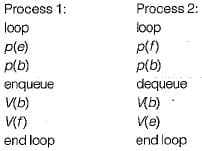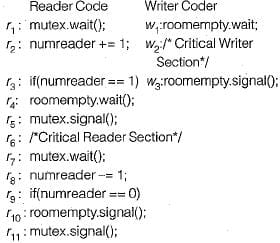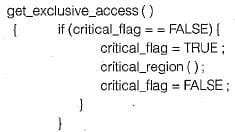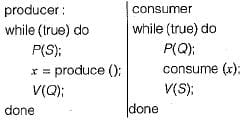# Test: IPC, Synchronization & Concurrency- 2

## 11 Questions MCQ Test GATE Computer Science Engineering(CSE) 2023 Mock Test Series | Test: IPC, Synchronization & Concurrency- 2

Description
Attempt Test: IPC, Synchronization & Concurrency- 2 | 11 questions in 30 minutes | Mock test for Computer Science Engineering (CSE) preparation | Free important questions MCQ to study GATE Computer Science Engineering(CSE) 2023 Mock Test Series for Computer Science Engineering (CSE) Exam | Download free PDF with solutions
QUESTION: 1

### At a particular time of computation, the value of a counting semaphore is 7. Then 20 P operation and ‘x’ V operation were completed on this semaphore. If the final value of the semaphore is 5, x will be

Solution:

Each P operation will decrease the semaphore value by 1 and V operation increases it by 1. If x is 18, then 7 Poperations will make semaphore value 0. If this is followed by 7 V operations the value comes back to 7. So, after 18 P and 18 V operations, the value of the semaphore will be 7. The remaining 2 P operations result in the semaphore values 5.

QUESTION: 2

### ‘Aging’ is

Solution:

‘Aging’ is a process that increases the priority of jobs corresponding to the time process is waiting for the CPU. So, the process with longer waiting time turns to have more priority over the process that is just arriving. This is done to ensure there is no STARVATION and termination of processes in a finite time.

QUESTION: 3

### Each process Pi, i = 1,2, 3,...., 9 is coded as follows: repeat P (mutex) { critical section } V (mutex) forever The code for P 10 is identical except that is uses V (mutex) instead of P (mutex). What is the largest number of processes that can be inside the critical section at any moment?

Solution:

Let the mutex be initialized to 1. Any one of the 9 processes Pi, i = 1 , 2 , 3 , ... 9 can get into the critical section after executing P (mutex) which decrements the mutex value to 0. At this time P10 can enter into the critical section as it uses V (mutex) instead of P(mutex) to get into the critical section. As a result of this, mutex will be incremented by 1. Now any one of the 9 processes Pi, i — 1, 2 , 3 , ... 9 (excepting the one that is already inside the critical section) can get into the critical section after decrementing the mutex to 0. None of the remaining processes can get into the critical section.
If the mutex is initialized to 0, only 2 processes can get into the critical section. So the largest number of processes is 3.

QUESTION: 4

Process P1 and P2 have a producer-consumer relationship, communicating by the use of a set of shared buffers:
P1 :
repeat
obtain an empty buffer
Fill it
return a full buffer
forever
P2:
repeat
obtain a full buffer
empty it
return an empty buffer
forever
Increasing the number of buffers is likely to do which of the following?
1. Increase the rate at which requests are satisfied.
2. Decrease the likelihood of deadlock.
3. Increase the case of achieving a correct implementation.

Solution:

Given set of processes depicts a standard producer-consumer relationship. Increasing the number of buffers will help in providing more slots to P1 to fill a buffer and more slots to P2 to empty a buffer. Hence, increase the rate at which requests are satisfied.
This will not affect the likelihood of deadlock:

QUESTION: 5

Consider a queue between the two processes indicated below. A/is the length of queue and e, f and b are semaphore, init : e : = N; f = 0; b : = 1;Which of the following statements is/are true?
1. The purpose of semaphore f is to ensure that dequeue is not executed on an empty queue.
2. The purpose of semaphore e is to ensure that deadlock does not occur.
​3. The purpose of semaphore b is to provide mutual exclusion for queue operations.

Solution:

e = N=> empty = N [The complete array is empty initially]
f= 0 = > full = 1 [No item is there in the array] b = 1 => Only one process can execute at a time

• The purpose of semaphore 'f' is to ensure that dequeue is not executed on an empty queue. Process 2 will block itself on first statement i.e. p(t).
• The purpose of semaphore ‘e’ is to ensure that not more than N elements being present in queue or if N element are already there in queue, process 1 must block itself.
• The purpose of semaphore ‘b’ is to ensure mutual exclusion for queue operations.
QUESTION: 6

Consider the following :
mutex = semaphore (1);
room empty (1);The above code is a proposed solution of the Readers/Writer problem.
Consider which of the following statements is/are true?
1.    The code allow several writers to execute in the writers critical area at the same time
2.    The code allow several readers to execute in the readers critical area at the same time.

Solution:
• Since binary semaphore used, so at a time either reader or writer can active inside critical section.
• Readsers can use binary semaphore without interversion of writers and can enter into critical section, so statement is true.
QUESTION: 7

Processes P1 and P2 use critical_flag in the following routine to achieve mutual exclusion. Assume that critical_flag is initialized to FALSE in the main program:1. It is possible for both P1 and P2 to access critical_region concurrently.
Which of the following holds?

Solution:

If after checking the condition (critical - flag == FALSE) P1 preempts without making critical flag TRUE and in turn gives option for P2 to check the condition and since critical - flag is FALSE till this point. P2 will also enter the if block and make the critical - flag as TRUE and start assessing critical -region ().
If P1 again comes back, it will start from time 2 directly and rewrite critical - flag as TRUE again and will start assessing critical - region (). So, it is possible for P1 and P2 to access critical - region concurrently.
Since, mutual exclusion is mandatory condition for deadlock, deadlock will never occur in this case. However, consistency can’t be ensured in such cases.

QUESTION: 8

The following is a code with two threads, producer and consumer, that can run parallel. Further, S and Q are binary semaphore equipped with the standards P and V operations,
semaphore S - 1, Q = 0
integer x;Which of the following is TRUE about the program above?

Solution:

Since there is a strict alteration between producer and consumer. So values generated and stored in 'x' by producer will always be consumed before the producer can generate a new value.

QUESTION: 9

Let R1, R2, R3 be reader processes and let W1 and W2 be writer processes requesting shared data. If R1 is selected for access. Which of the statement is/are correct?
1. Mutual exclusion is necessary for R2 and R3.
2. No mutual exclusion is necessary for R2 and R3.
3. Mutual exclusion must be there for W1 and W2.
4. No mutual exclusion must be there for W1 and W2.

Solution:

If R1 accesses the critical section:
1. No mutual exclusion needed for R2 and R3.
2, Mutual exclusion must be there for W1and W2.

QUESTION: 10

Assume that 'C' is counting semaphore. Consider the following program segment:
C= 10;
P(C);
V(C);
P(C);
P(C);
P(C);
V(C);
P(C);
P(C);
What is the value of 'C' at the end of the above program execution?

Solution:

Number of P(C) wait process = 6 i.e. decrement

Number of V(C) signal process = 2 i.e. increment

Therefore value of ‘C’ at the end of program execution
= Initial value - Number of P(C) + Number of V(C)
= 10 - 6 + 2 = 6

QUESTION: 11

At a particular time of ocmputation the value of a counting semaphore is 7. Then 20 P operations and 15 V operation were completed on this semaphore. The resulting value of the semaphore is

Solution:

Number of wait process = 20 i.e. decrement Number of signal process = 15 i.e. increment Therefore value of semaphore at the end of program execution
= Initial value - Number of P(C) + Number of V(C)
= 7 - 20 + 15 = 2Use Code STAYHOME200 and get INR 200 additional OFF Use Coupon Code